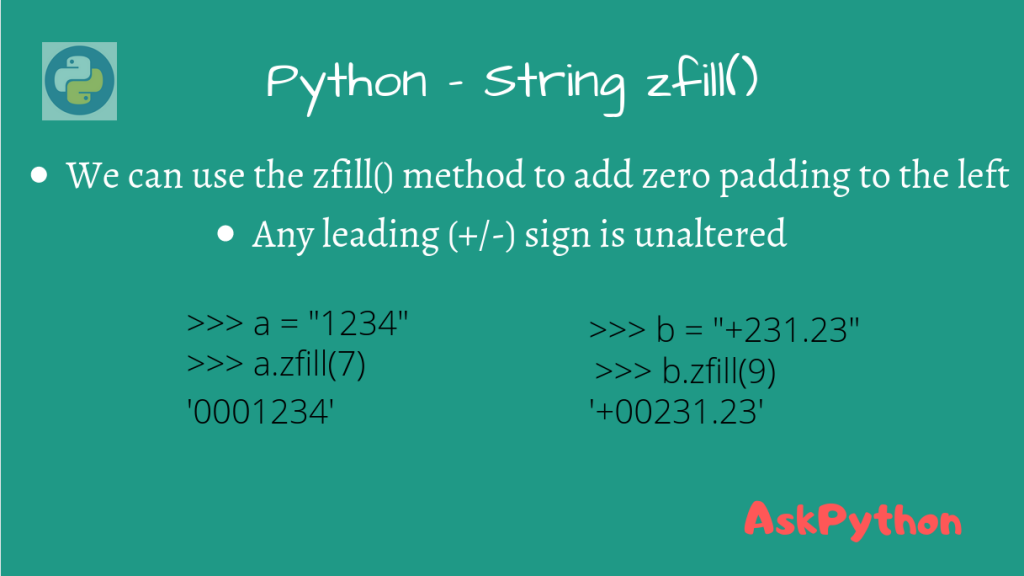# Python string zfill()The Python string zfill() method is used to pad a string with zeros on the left until a specific width is reached. This is the most “Pythonic” way to add padding to a string, instead of manually iterating using for loops.

## Syntax of Python String zfill() Method

The Python string zfill() function belongs to the String class, and can only be used on string objects.

This takes in width as a parameter, and pads zeros on a string’s left (start of the string) till the specified width is reached.

If width < len(str), then the original string is returned. Otherwise, since Python strings are immutable, this function returns a new string.

```new_str = str.zfill(width)
```

If there is a leading sign (+/-), the padding is added after the sign, maintaining the position of the leading sign even in the new string.

Let’s look at some examples now.

If there is no leading sign, the zeros are padded to the left.

```>>> a = "AskPython"
>>> a.zfill(15)
```

As you can see, the zeros are padded to the left, and the length of the new string is 15.

If the specified width is less than len(“AskPython”), then we get the original string.

```>>> a = "AskPython"
>>> a.zfill(len(a) - 1)
>>> a.zfill(len(a) - 1) == a
True
```

If there is a leading +/- sign, the zeros are padded after the sign.

```>>> a = "+123456"
>>> a.zfill(len(a) - 1)
'+123456'
>>> a.zfill(10)
'+000123456'

>>> a = "-123456"
>>> a.zfill(10)
'-000123456'
```

## Alternatives to Python String zfill()

Besides zfill(), we can use a couple of other methods to introduce zero paddings in a string.

### Using str.rjust()

Similar to str.zfill(), the str.rjust() can also give padding to a string, but also allows us to specify the character to pad the string with. Have a look at the example below.

```>>> a = "AskPython"
>>> a.rjust(15, "0")
>>> a.rjust(15, "1")

```

Here, we introduced zero-padding using `rjust(width, '0')` and one padding using `rjust(width, '1')`. You can replace the numbers with any character.

### Using Print String Formatting

In case you’re working with real numbers, you can also use Python f-strings (from Python 3.6 and above) to return a zero-padded string.

```>>> a = 1.414
>>> print(f'{a:013}')
'000000001.414'
```